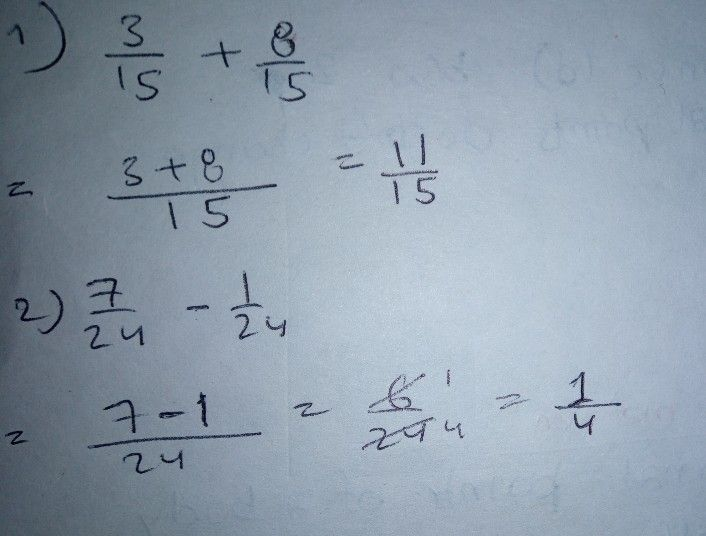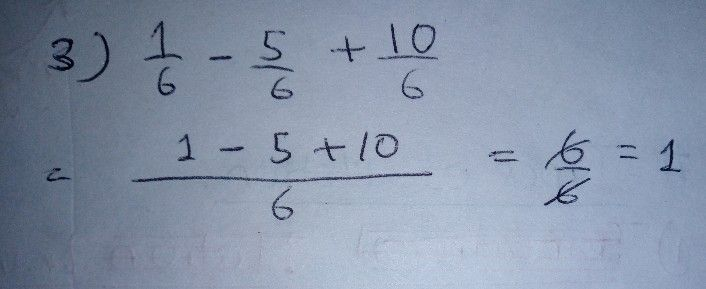Symbol
ProblemB. Directions. P'erform the indicated operations and reduce your answers to the lowest form. Write your answers on a separate sheet of paper. $1.\dfrac {3} {15}+\dfrac {8} {15}$ $2$ $\dfrac {7} {24}-\dfrac {1} {24}$ $3.\dfrac {1} {5}-\dfrac {5} {6}+$ $\dfrac {1} {6}-\dfrac {5} {6}+\dfrac {10} {6}$
7th-9th grade
Algebra
Search count: 115
SolutionQanda teacher - Chris